##### Machine Learning For Dummies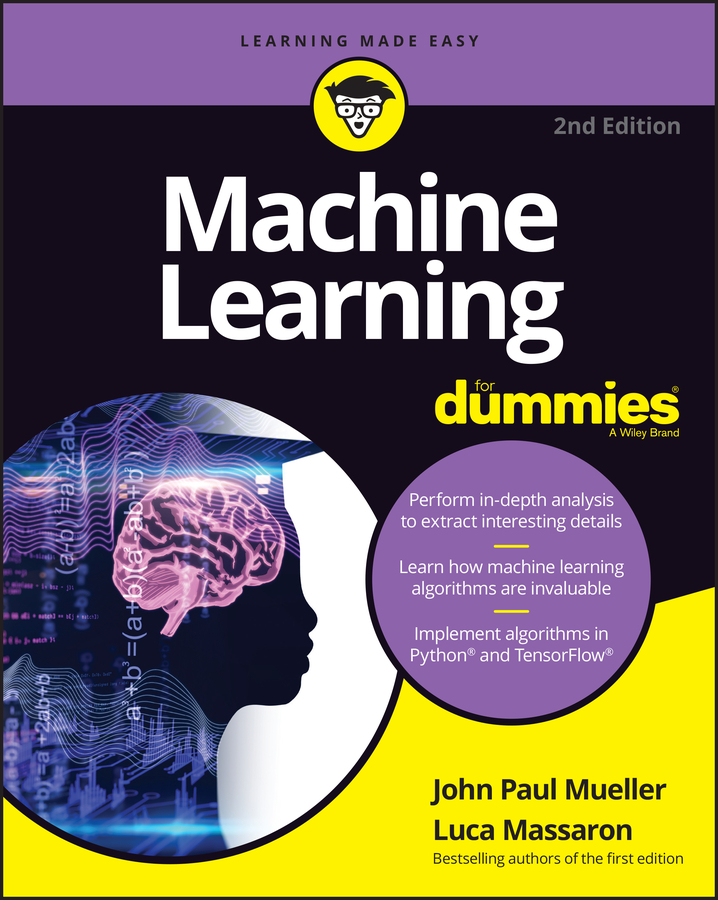Even though supervised learning is the most popular and frequently used of the three types, all machine learning algorithms respond to the same logic. The central idea is that you can represent reality using a mathematical function that the algorithm doesn’t know in advance but can guess after having seen some data. You can express reality and all its challenging complexity in terms of unknown mathematical functions that machine learning algorithms find and make advantageous. This concept is the core idea for all kinds of machine learning algorithms.

To create clear examples, this article focuses on supervised classification as the most emblematic of all the learning types and provides explanations of its inner functioning that you can extend later to other types of machine learning approaches.

The objective of a supervised classifier is to assign a class (also called a label) to an example after having examined some characteristics of the example itself. Such characteristics are called features, and they can be either quantitative (numeric values) or qualitative (nonnumeric values such as string labels). To assign classes correctly, the classifier must first closely examine a certain number of known examples (examples that already have a class assigned to them), each one accompanied by the same features. This learning procedure, also called the training phase, involves observation of many examples and their labels by the classifier that helps it learn so that it can provide an answer in terms of a class when it sees an example without a class later at prediction time.

Both the data that you use for the training phase and the data that you use for making new predictions using your trained model (the phase called testing), should share the exact same features you used during training or the predictions won’t work correctly.

## Mapping an unknown function

To give an idea of what happens in the training process, imagine a child learning to distinguish trees from other objects. Before the child can do so in an independent fashion, a teacher presents the child with a certain number of tree images, complete with all the facts that make a tree distinguishable from other objects of the world. Such facts could be features such as its material (wood), its parts (trunk, branches, leaves or needles, roots), and location (planted into the soil). The child produces an idea of what a tree looks like by contrasting the display of tree features with the images of other different objects, such as pieces of furniture that are made of wood but do not share other characteristics with a tree.

A machine learning classifier works the same. It builds its cognitive capabilities by creating a mathematical formulation that includes all the given features in a way that creates a function that can distinguish one class from another. Pretend that a mathematical formulation, also called a target function (or an objective function), exists to express the characteristics of a tree. In such a case, a machine learning classifier can look for the representation of the target function as a replica or as an approximation (a different function that works alike). Being able to express such mathematical formulation is the representation capability of the classifier.

From a mathematical perspective, you can express the representation process in machine learning using the equivalent term mapping. Mapping happens when you discover the construction of a function by observing its outputs. A successful mapping in machine learning is similar to a child internalizing the idea of an object. In this case, the child understands the abstract rules derived from the facts of the world in an effective way so that it’s possible to recognize a tree when seeing one.

Such a representation (abstract rules derived from real-world facts) is possible because the learning algorithm has many internal parameters (constituted of vectors and matrices of values), which equate to the algorithm’s memory for ideas that are suitable for its mapping activity that connects features to response classes. The dimensions and type of internal parameters delimit the kind of target functions that an algorithm can learn. An optimization engine in the algorithm changes parameters from their initial values during learning to represent the target’s hidden function.

During optimization, the algorithm searches among possible variants of its parameter combinations to find the best combination that allows correct mapping between features and classes during training. This process evaluates many potential candidate target functions from among those that the learning algorithm can guess. The set of all the potential functions the learning algorithm can evaluate is the hypothesis space. You can call the resulting classifier with all its set parameters a hypothesis, a way in machine learning to say that the algorithm has set parameters to replicate the target function and is now ready to work out correct classifications.

The hypothesis space must contain all the parameter variants of all the machine learning algorithms that you want to try to map to an unknown function when solving a classification problem. Different algorithms can have different hypothesis spaces. What really matters is that the hypothesis space contains the target function (or its approximation, which is a different but similar function).

You can imagine this phase as the time when a child, in an effort to figure out the idea of a tree, experiments with many different creative ideas by assembling knowledge and experiences (an analogy for the given features). Naturally, the parents are involved in this phase, and they provide relevant environmental inputs. In machine learning, someone has to provide the right learning algorithms, supply some nonlearnable parameters (called hyper-parameters), choose a set of examples to learn from, and select the features that accompany the examples. Just as a child can’t always learn to distinguish between right and wrong if left alone in the world, so machine learning algorithms need human beings to learn successfully.

Even after completing the learning process, a machine learning classifier often can’t univocally map the examples to the target classification function because many false and erroneous mappings are also possible, as shown.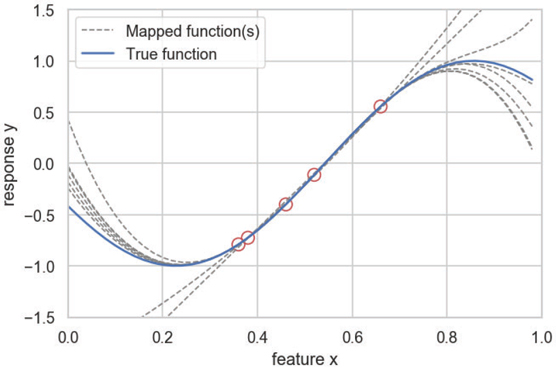A lack of evidence makes it hard to map back to the target function.

In many cases, the false and erroneous mappings occur because the algorithm lacks enough data points to discover the right function. Noise, erroneous or distorted examples, mixed with correct data can also cause problems, as shown.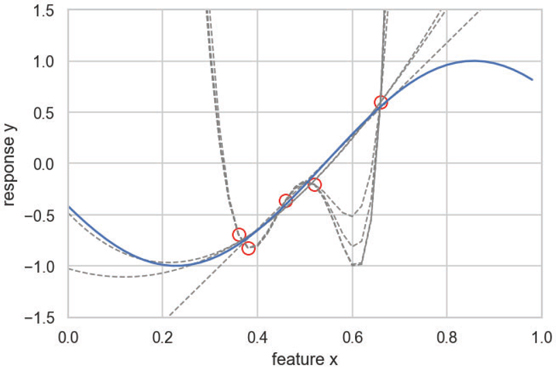Noise can cause mismatches in the data points.

Noise in real-world data is the norm. Many extraneous factors and errors that occur when recording data distort the values of the features. A good machine learning algorithm should distinguish the signals that can map back to the target function and ignore extraneous noise.

## Cost functions

The driving force behind optimization in machine learning is the response from a function internal to the algorithm, called the cost function. You may see other terms used in some contexts, such as loss function, objective function, scoring function, or error function, but the cost function is an evaluation function that measures how well the machine learning algorithm maps the target function that it’s striving to guess. In addition, a cost function determines how well a machine learning algorithm performs in a supervised prediction or an unsupervised optimization problem (in this latter case, the cost function is not related to the target outcome but to the features themselves).

The cost function works by comparing the algorithm predictions against the actual outcome recorded from the real world. Comparing a prediction against its real value using a cost function determines the algorithm’s error level. Because it’s a mathematical formulation, the cost function expresses the error level in a numerical form, a cost value that has to be minimized. The cost function transmits what is actually important and meaningful for your purposes to the learning algorithm. As a result, you must choose, or accurately define, the cost function based on an understanding of the problem you want to solve or the level of achievement you want to reach.

As an example, when considering stock market forecasting, the cost function expresses the importance of avoiding incorrect predictions. In this case, you want to make money by avoiding big losses. In forecasting sales, the concern is different because you need to reduce the error in common and frequent situations, not in the rare and exceptional ones, so you use a different cost function.

When the problem is to predict who will likely become ill from a certain disease, you prize algorithms that can score a high probability of singling out people who have the same characteristics and actually did become ill later. Based on the severity of the illness, you may also prefer that the algorithm wrongly chooses some people who don’t get ill, rather than miss the people who actually do get ill.

The cost function is what truly drives the success of a machine learning application. It’s as critical to the learning process as representation (the capability to approximate certain mathematical functions) and optimization (how the machine learning algorithms set their internal parameters). Most algorithms optimize their own cost function, and you have little choice but to apply them as they are. Some algorithms allow you to choose among a certain number of possible functions, providing more flexibility. When an algorithm uses a cost function directly in the optimization process, the cost function is used internally. Given that algorithms are set to work with certain cost functions, the optimization objective may differ from your desired objective. In such a case, you measure the results using an external cost function that, for clarity of terminology, you call an error function or loss function (if it has to be minimized) or a scoring function (if it has instead to be maximized).

With respect to your target, a good practice is to define the cost function that works the best in solving your problem, and then to figure out which algorithms work best in optimizing it to define the hypothesis space you want to test. When you work with algorithms that don’t allow the cost function you want, you can still indirectly influence their optimization process to fit your preferred cost function by fixing their hyper-parameters (the parameters that you have to provide for the algorithm to work) and selecting your input features with respect to your cost function. Finally, when you’ve gathered all the results from the algorithms, you evaluate them by using your chosen cost function and then decide what mix of algorithm, hyper-parameters, and features is the best to solve your problem.

When an algorithm learns from data, the cost function guides the optimization process by pointing out the changes in the internal parameters that are the most beneficial for making better predictions. The optimization continues as the cost function response improves iteration by iteration. When the response stalls or worsens, it’s time to stop tweaking the algorithm’s parameters because the algorithm isn’t likely to achieve better prediction results from there on. When the algorithm works on new data and makes predictions, the cost function helps you evaluate whether it’s working properly and is indeed effective.

Deciding on the cost function is an underrated activity in machine learning. It’s a fundamental task because it determines how the algorithm behaves during the learning phase and how it handles the problem you want to solve. Never rely on default options, but always ask yourself what you want to achieve using machine learning and check what cost function can best represent the achievement.

## Descending the optimization curve

The gradient descent algorithm offers a perfect example of how machine learning works. Though it is just one of many possible methods, gradient descent is a widely used approach that’s applied to a series of machine learning algorithms, such as linear models, neural networks, and gradient boosting machines.

Gradient descent works out a solution by starting from a random solution when given a set of inputs (a data matrix made of features and a response). It then proceeds in various iterations using the feedback from the cost function, thus changing its parameters with values that gradually improve the initial random solution and lower the error. Even though the optimization may take a large number of iterations before reaching a good mapping, it relies on changes that improve the response cost function the most during each iteration. This figure shows an example of a complex optimization process with some local minima (the minimum points at the middle of the valleys) and a place where the process can get stuck (because of the flat surface at the saddle point) and cannot continue its descent.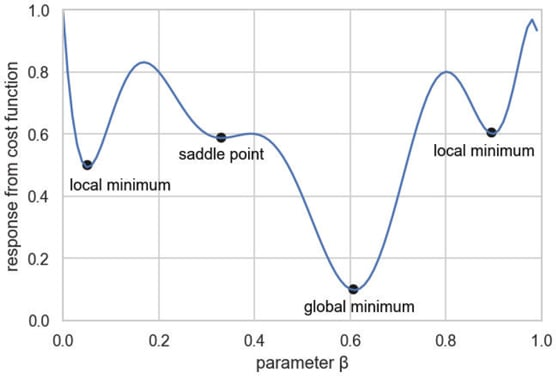A plotting of parameter data against the output of the cost function.

Based on this figure, you can visualize the optimization process as a walk in high mountains, during a misty day, with the parameters being the different paths to descend to the valley. A gradient descent optimization occurs at each step. At each iteration, the algorithm chooses the path that reduces error the most, regardless of the direction taken. The idea is that if steps aren’t too large (causing the algorithm to jump over the target), always following the most downward direction will result in finding the lowest place.

Unfortunately, finding the lowest place doesn’t always occur because the algorithm can arrive at intermediate valleys, creating the illusion that it has reached the target. However, in most cases, gradient descent leads the machine learning algorithm to discover the right hypothesis for successfully mapping the problem. This figure shows how a different starting point can make the difference. Starting point A ends toward a local minimum, whereas not far away point B manages to reach the global minimum.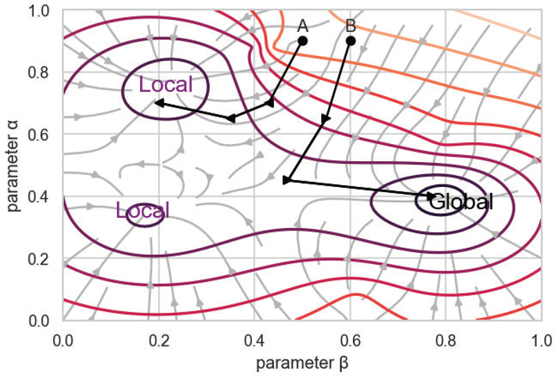Visualizing the effect of starting point on outcome.

In an optimization process, you distinguish between different optimization outcomes. You can have a global minimum that’s truly the minimum error from the cost function, and you can have many local minima—solutions that seem to produce the minimum error but actually don’t (the intermediate valleys where the algorithm gets stuck). As a remedy, given the optimization process’s random initialization, running the optimization many times is good practice. This means trying different sequences of descending paths and not getting stuck in the same local minimum.